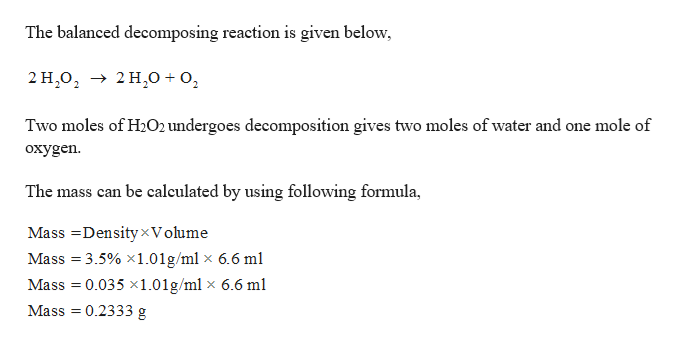# The catalytic decomposition of hydrogen peroxide can be expressed as:2H2O2(aq) → 2H2O(l) + O2(g)Calculate the number of moles of oxygen gas produced from the completely catalyzed decomposition of 6.60 mL sample of a 3.5% solution of H2O2. The density of the 3.5% solution of H2O2 is 1.01 g/mL.

Question
48 views

The catalytic decomposition of hydrogen peroxide can be expressed as:

2H2O2(aq) → 2H2O(l) + O2(g)

Calculate the number of moles of oxygen gas produced from the completely catalyzed decomposition of 6.60 mL sample of a 3.5% solution of H2O2. The density of the 3.5% solution of H2O2 is 1.01 g/mL.

check_circle

Step 1

given,

Density = 3.5 %

Volume = 6.60 ml

Molar mass of H2...help_outlineImage TranscriptioncloseThe balanced decomposing reaction is given below 2 Н.о, > 2 H,о + о, Two moles of H2O2 undergoes decomposition gives two moles of water and one mole of охygen. The mass can be calculated by using following formula Mass DensityxV olume Mass 3.5% x1.01g/ml x 6.6 ml Mass 0.035 x1.01g/ml x 6.6 ml Mass 0.2333 g fullscreen

### Want to see the full answer?

See Solution

#### Want to see this answer and more?

Solutions are written by subject experts who are available 24/7. Questions are typically answered within 1 hour.*

See Solution
*Response times may vary by subject and question.
Tagged in

### General Chemistry